# Corrective Math 2005

Grade Levels: 3-13
Corrective Mathematics provides intensive support for students who have difficulty with mathematics. The series is organized into seven strategic modules that provide teacher-directed instruction on critical skills and concepts which struggling students often fail to grasp.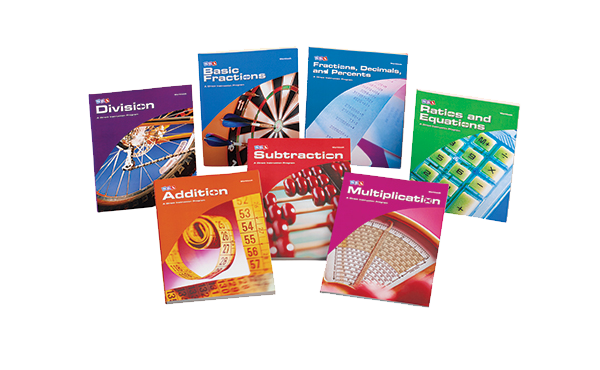## About the Program

Corrective Mathematics is a highly focused, intervention program consisting of seven modules that offer quick, targeting intervention to build understanding of:

• Addition
• Subtraction
• Multiplication
• Division
• Basic Fractions
• Fractions, Decimals, and Percents
• Ratios and Equations

By focusing on core content and breaking this content into a series of small conceptual steps and embedded skills, Corrective Mathematics enables you to teach mathematics skills, rules, and strategies efficiently and effectively. It ensures that students will learn to work mathematics problems accurately and confidently.

The series is not intended to serve as a fixed course of study. Instead it is used to support a high-quality core mathematics program. A placement test provides a plan that identifies which modules need to be covered.

Student Materials:

• Workbooks include daily worksheets and point summary charts for recording student performance and awarding grades.

Teacher Materials include:

• Presentation Books include a Guide section containing information for presenting exercises, correcting mistakes, and administering the pre-skill and placement tests. There is also a Presentation section that contains detailed lesson plans.
• Answer Key Booklets quickly and easily compare students' work with the actual calculations and word problem results.

Additional Resources:

• ExamView Software provides dynamic worksheets and creates paper- or computer-based tests and worksheets (available in 2 formats - ExamView for Addition, Subtraction, Multiplication, Division AND ExamView for Basic Fractions; Fractions, Decimals, and Percents; and Ratios and Equations).
• Additional Answer Key Booklets make it easy to check students' independent work.
• Series Guide helps determine what module your students are in by administering the pre-skill and placement tests. Guide also contains sample lessons from each of the seven modules.

## Program Components

•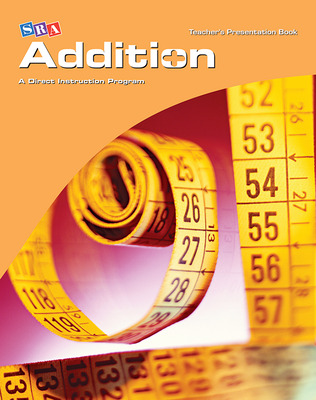Corrective Mathematics Addition, Teacher Materials
•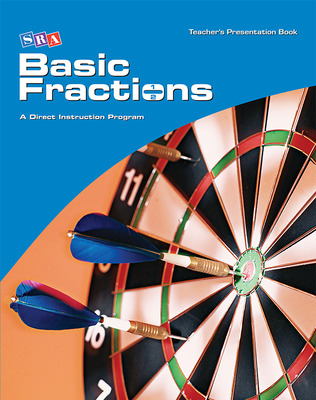Corrective Mathematics Basic Fractions, Teacher Materials
•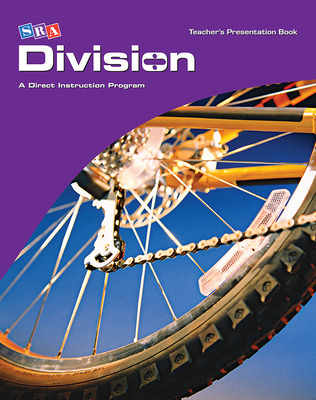Corrective Mathematics Division, Teacher Materials
•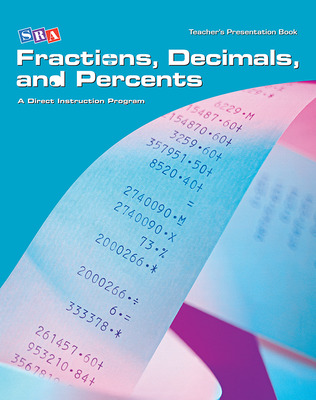Corrective Mathematics Fractions, Decimals, and Percents, Teacher Materials
•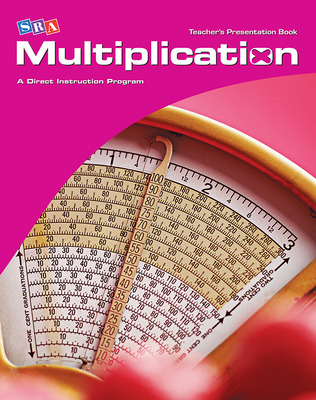Corrective Mathematics Multiplication, Teacher Materials
•Corrective Mathematics Ratios and Equations, Additional Answer Key
•Corrective Mathematics Ratios and Equations, Teacher Material
•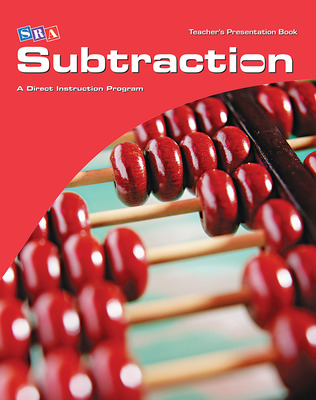Corrective Mathematics Subtraction, Teacher Materials
•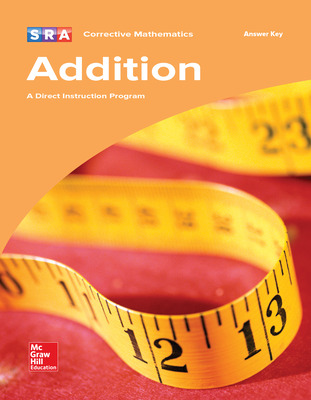Corrective Mathematics Addition, Additional Answer Key
•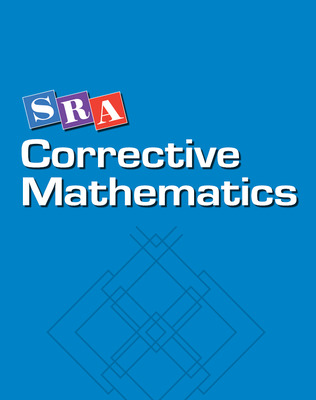Corrective Mathematics Addition, Subtraction, Multiplication, Division, ExamView Local Area Network (LAN) Version
•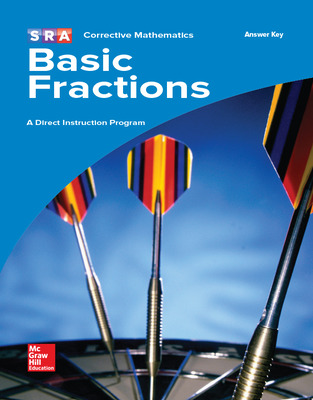Corrective Mathematics Basic Fractions, Additional Answer Key
•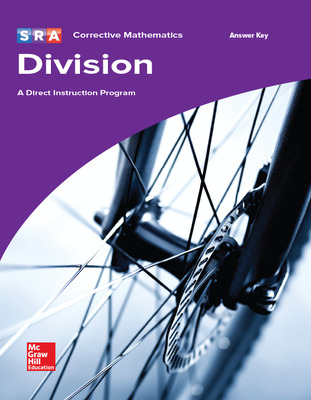Corrective Mathematics Division, Additional Answer Key
•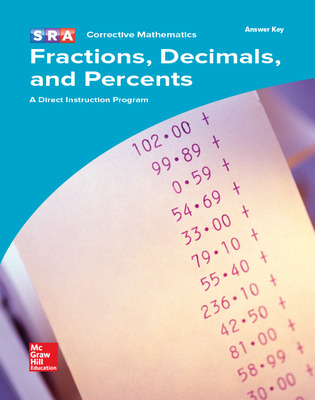Corrective Mathematics Fractions, Decimals, and Percents, Additional Answer Key
•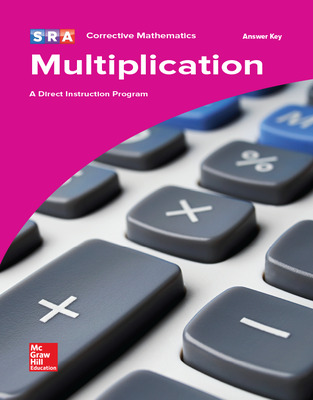Corrective Mathematics Multiplication, Additional Answer Key
•Corrective Mathematics Ratios and Equations, Teacher Presentation Book
•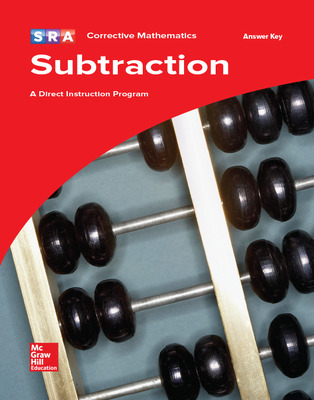Corrective Mathematics Subtraction, Additional Answer Key
•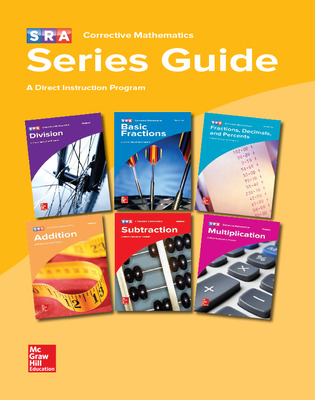Corrective Mathematics, Series Guide
•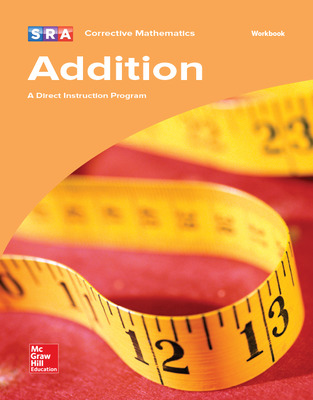Corrective Mathematics Addition, Workbook
•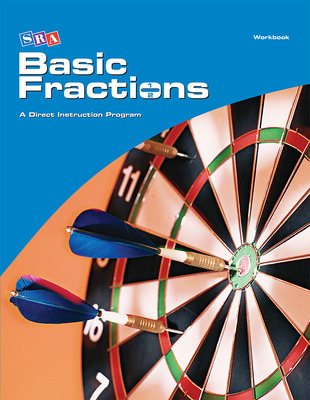Corrective Mathematics Basic Fractions, Workbook
•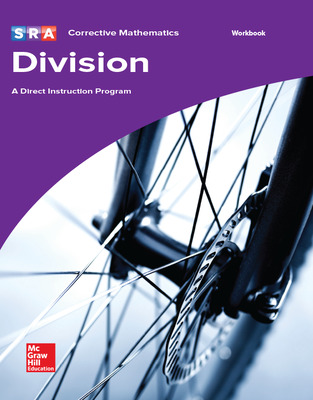Corrective Mathematics Division, Workbook
•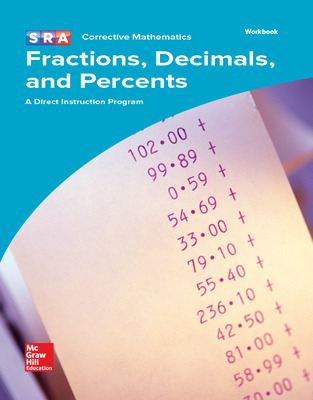Corrective Mathematics Fractions, Decimals, and Percents, Workbook
•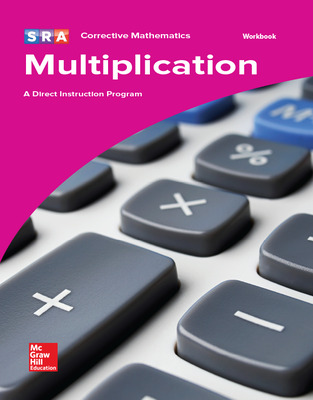Corrective Mathematics Multiplication, Workbook
•Corrective Mathematics Ratios and Equations, Workbook Package of 5
•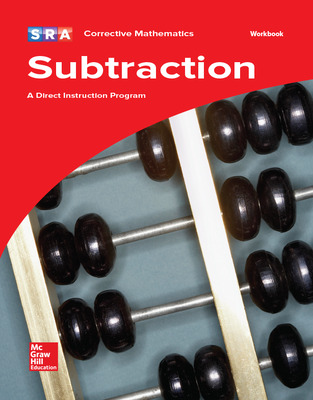Corrective Mathematics Subtraction, Workbook
Sort by:

## Products

To learn about the specific purchase options for this program, please contact our Customer Service team at 800-338-3987

## Correlations

Research Base

• Direct Instruction Mathematics Programs: An Overview and Research Summary
This paper provides an overview and research summary of Direct Instruction (DI) mathematics programs, including Connecting Math Concepts . A comparison of the constructivist approach to the direct or explicit approach to math instruction was conducted. Overviews and ways in which DI math programs meet the 6 principles for improving math instruction as provided by the National Council of Teachers of Mathematics (NCTM; 2000b) are noted. Finally, a research review and analysis of DI math programs published since 1990 (yielding 12 studies) was completed.
• The Research Base for Direct Instruction Mathematics Programs
The following is a description of the research base for Direct Instruction mathematics programs, specifically DISTAR Arithmetic I and II, Corrective Mathematics, and Connecting Math Concepts. Searched of reference lists were used to identify other possible research articles. Also, hand searches were done in the following peer-reviewed journals: Effective School Practices, Journal of Direct Instruction, and Educations and Treatment of Children.
• Mathematics Education for Students with Learning Disabilities
The Center on Instruction conducted a meta-analysis on the topic of teaching mathematics to students with learning disabilities (Gersten, Chard, Jayanthi, Baker, Morphy, & Flojo, 2008). A meta-analysis is a statistical method by which research studies on a particular method of instruction are summarized to determine the effectiveness of that instructional method. Based on the findings of the meta-analysis report, seven effective instructional practices were identified for teaching mathematics to K–12 students with learning disabilities.

Randomized Controlled Studies

Non-Randomized Controlled Studies

Evidence of Success

• Special Education and Direct Instruction: An Effective Combination
Research shows strong evidence of success when Direct Instruction programs are used with students with special needs. In fact, Direct Instruction is one of only seven interventions proven effective (Forness, Kavale, Blum & Lloyd, 1997). With its research-supported design and systematic delivery, Direct Instruction is often referred to as a program for special education or at-risk students.